# Theorem Network from Euclid's Elements

Graph of interdependence of theorems from Euclid's Elements

## Details

The nodes correspond to theorems, common notions and postulates.
Each node is a connected to nodes corresponding to the theorems etc. referenced in its proof.
A version of this network originally appeared in A New Kind of Science (Wolfram, 2002) on page 1176.

## Examples

### Basic Examples

Retrieve the basic network:

 In:=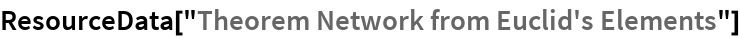Out=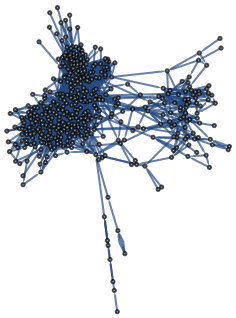Make a layered graph plot:

 In:=Out=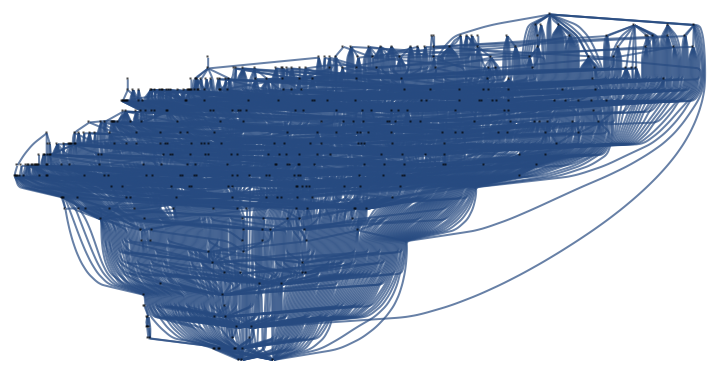Find axioms and theorems referenced by Book 1, Theorem 5:

 In:=Out=### Analysis

Count the total number of theorems + axioms:

 In:=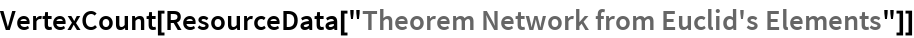Out=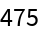Find the axioms:

 In:=Out=Find the distribution of numbers of theorems referenced:

 In:=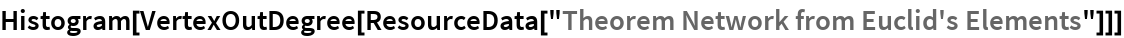Out=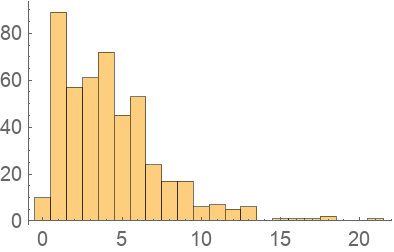Find the 5 most referenced theorems:

 In:=Out=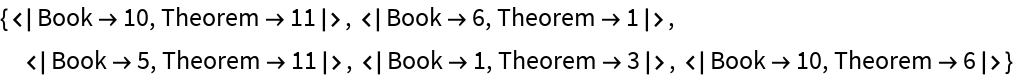Find the total number of theorems + axioms that ultimately contribute to the proof of each theorem:

 In:=Out=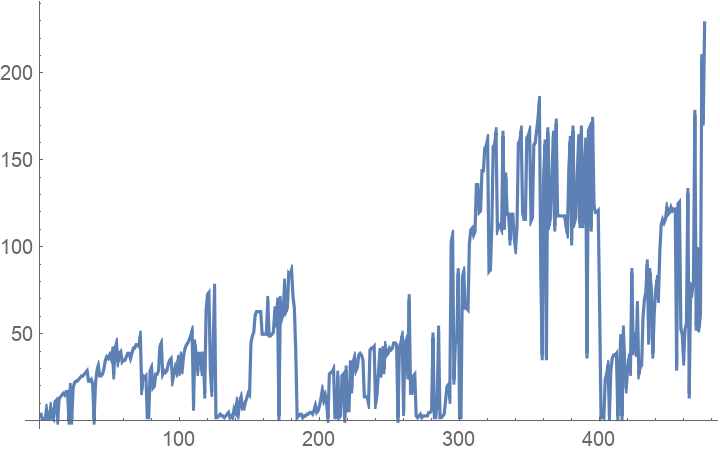Create a key of axiom + theorem number:

 In:=Make a map of what theorem references which others:

 In:=Out=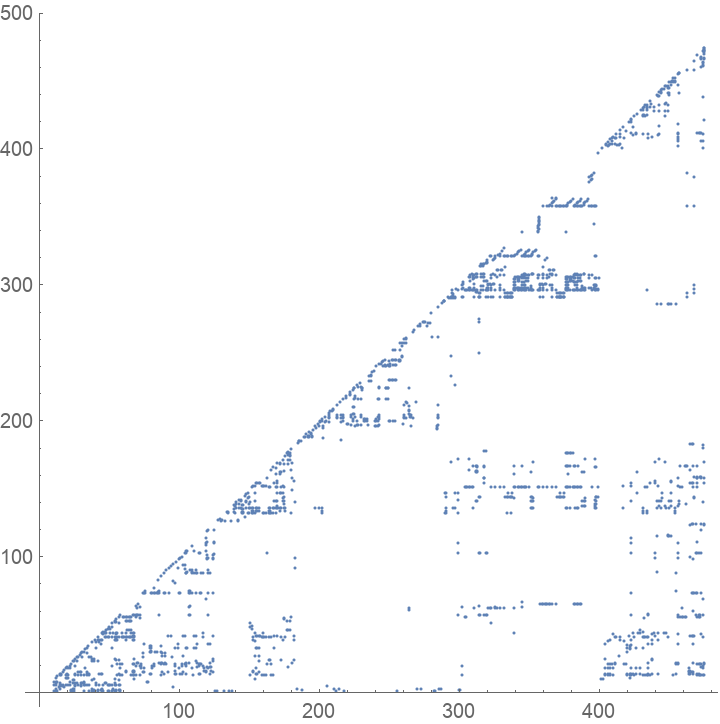Wolfram Research, "Theorem Network from Euclid's Elements" from the Wolfram Data Repository (2020) https://doi.org/10.24097/wolfram.61787.data